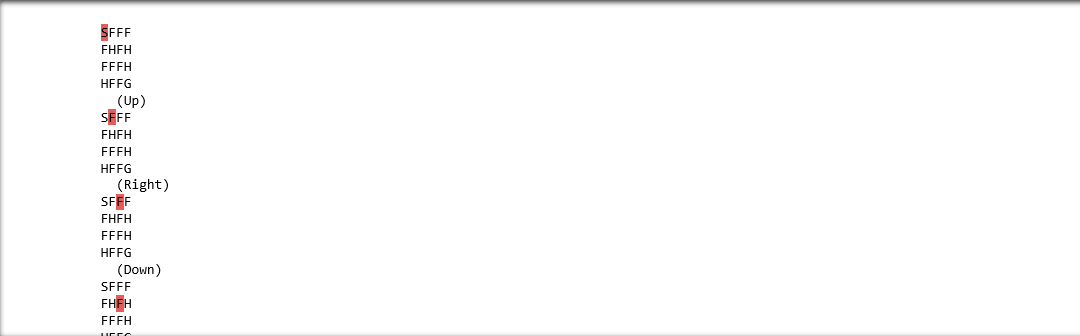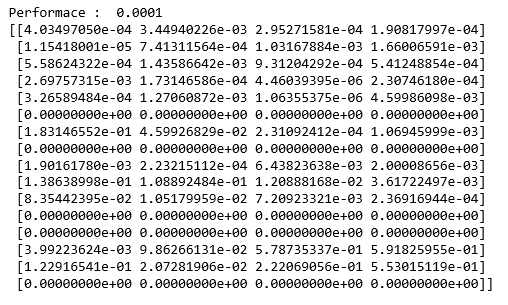# SARSA Reinforcement Learning

Prerequisites: Q-Learning technique

SARSA algorithm is a slight variation of the popular Q-Learning algorithm. For a learning agent in any Reinforcement Learning algorithm it’s policy can be of two types:-

1. On Policy: In this, the learning agent learns the value function according to the current action derived from the policy currently being used.
2. Off Policy: In this, the learning agent learns the value function according to the action derived from another policy.

Q-Learning technique is an Off Policy technique and uses the greedy approach to learn the Q-value. SARSA technique, on the other hand, is an On Policy and uses the action performed by the current policy to learn the Q-value.

This difference is visible in the difference of the update statements for each technique:-

1. Q-Learning:2. SARSA:Here, the update equation for SARSA depends on the current state, current action, reward obtained, next state and next action. This observation lead to the naming of the learning technique as SARSA stands for State Action Reward State Action which symbolizes the tuple (s, a, r, s’, a’).

The following Python code demonstrates how to implement the SARSA algorithm using the OpenAI’s gym module to load the environment.

Step 1: Importing the required libraries

 import numpy as np  import gym

Step 2: Building the environment

Here, we will be using the ‘FrozenLake-v0’ environment which is preloaded into gym. You can read about the environment description here.

 #Building the environment  env = gym.make('FrozenLake-v0')

Step 3: Initializing different parameters

 #Defining the different parameters  epsilon = 0.9 total_episodes = 10000 max_steps = 100 alpha = 0.85 gamma = 0.95    #Initializing the Q-matrix  Q = np.zeros((env.observation_space.n, env.action_space.n))

Step 4: Defining utility functions to be used in the learning process

 #Function to choose the next action  def choose_action(state):      action=0     if np.random.uniform(0, 1) < epsilon:          action = env.action_space.sample()      else:          action = np.argmax(Q[state, :])      return action     #Function to learn the Q-value  def update(state, state2, reward, action, action2):      predict = Q[state, action]      target = reward + gamma * Q[state2, action2]      Q[state, action] = Q[state, action] + alpha * (target - predict)

Step 5: Training the learning agent

 #Initializing the reward  reward=0    # Starting the SARSA learning  for episode in range(total_episodes):      t = 0     state1 = env.reset()      action1 = choose_action(state1)         while t < max_steps:          #Visualizing the training          env.render()                     #Getting the next state          state2, reward, done, info = env.step(action1)             #Choosing the next action          action2 = choose_action(state2)                     #Learning the Q-value          update(state1, state2, reward, action1, action2)             state1 = state2          action1 = action2                     #Updating the respective vaLues          t += 1         reward += 1                    #If at the end of learning process          if done:              breakIn the above output, the red mark determines the current position of the agent in the environment while the direction given in brackets gives the direction of movement that the agent will make next. Note that the agent stays at it’s position if goes out of bounds.

Step 6: Evaluating the performance

 #Evaluating the performance  print ("Performace : ", reward/total_episodes)     #Visualizing the Q-matrix  print(Q)My Personal Notes arrow_drop_upCheck out this Author's contributed articles.

If you like GeeksforGeeks and would like to contribute, you can also write an article using contribute.geeksforgeeks.org or mail your article to contribute@geeksforgeeks.org. See your article appearing on the GeeksforGeeks main page and help other Geeks.

Please Improve this article if you find anything incorrect by clicking on the "Improve Article" button below.

Article Tags :
Practice Tags :

1

Please write to us at contribute@geeksforgeeks.org to report any issue with the above content.